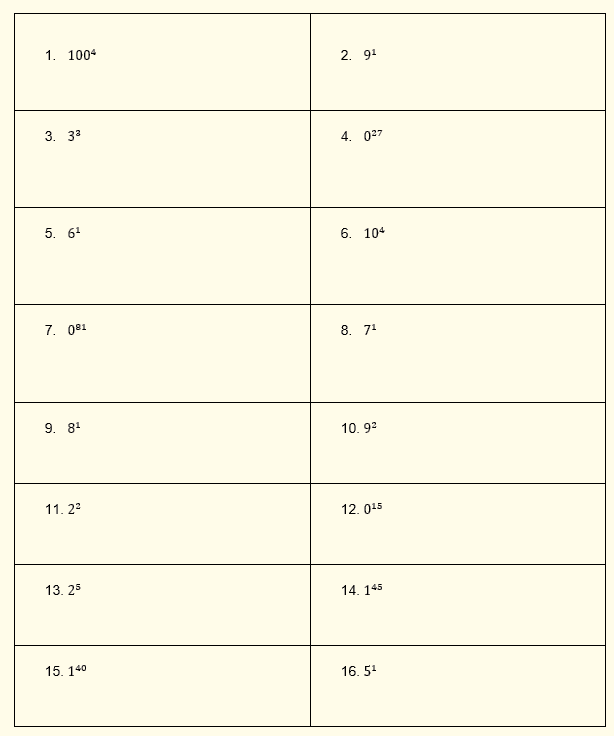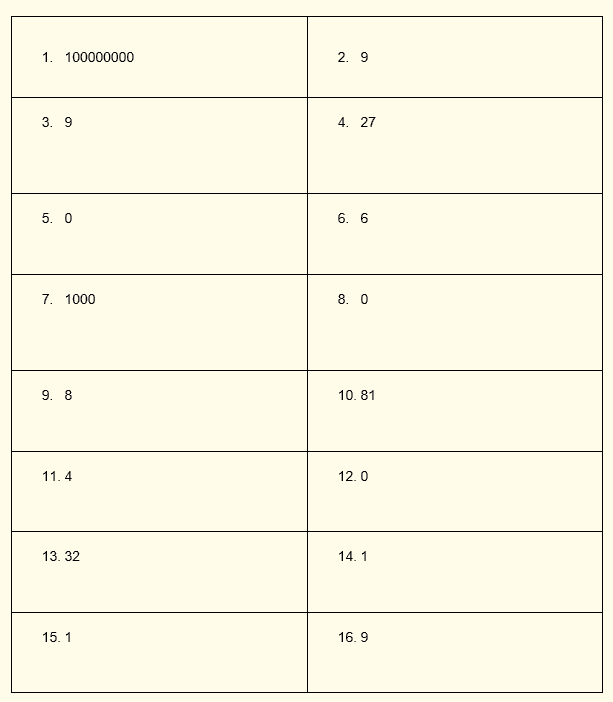A worksheet on exponents for the 7th grade is a great way to assess students’ understanding of math vocabulary and reasoning. For students, this worksheet offers a great introduction to pre-algebra skills. With the aid of straightforward step-by-step worksheets, these worksheets make exponents simpler.

This worksheet provides an understanding of math vocabulary and reasoning.

## What is Exponent?

Exponent: The tiny number to the right-hand side of another number. You can find out how many times the base value has been multiplied by itself using an exponent.

This worksheet will assist students in navigating the exponentiation world. Exponents worksheets for the seventh-grade help students and teach them strategies that will help them in more difficult math.

Instructions on how to use the “Exponents Worksheet Grade 7 (with answers).”

The students are given a few questions to answer after studying the concept and provided examples in order to put what they have learned about exponents into practice.

## Conclusion

Exponent is a symbol used to denote the operation of raising to a power and written above and to the right of a mathematical expression. At the end of this lesson, the students are able to familiarize themselves with the use of exponents.

If you have any inquiries or feedback, please let us know.

The exponent is the small number that is located at the top right of another number. The base is the large number that is at the bottom left of an exponent. A symbol is written above and to the right of a mathematical expression to indicate the operation of raising a power.

Example A: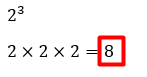Example B: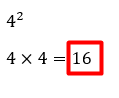Remember: 23 and 42 are not the same as this 2 x 3 and 4 x 2.

There are some special cases:

• When an exponent is zero, as in  60, the expression is always equal to 1.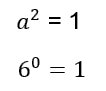• There is always a fraction as a result when the exponent is negative. A numerator and a denominator are the components of fractions. The numerator in this case is consistently 1. Pretend that the exponent is positive even though it is negative and raise the number to that power to find the denominator as in the following example: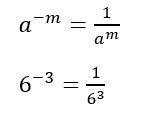## Solve.